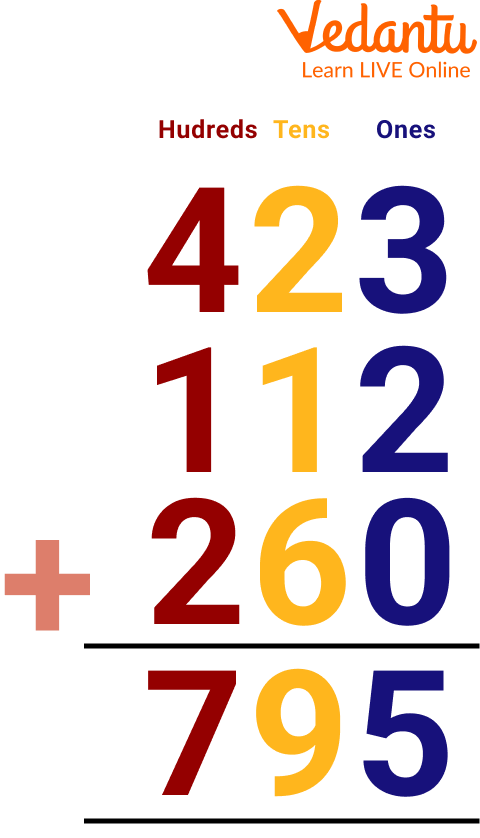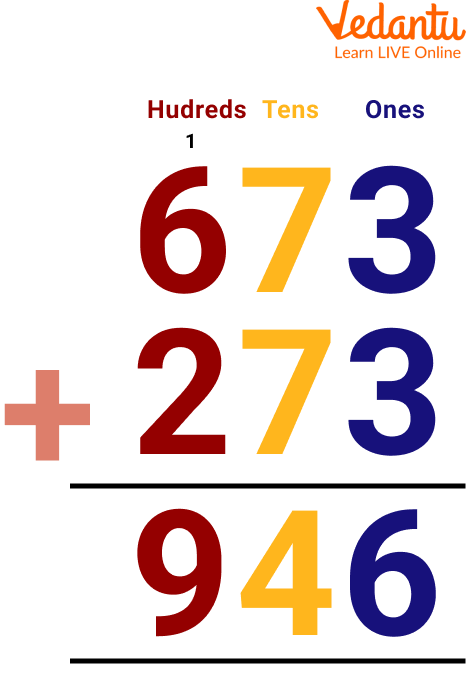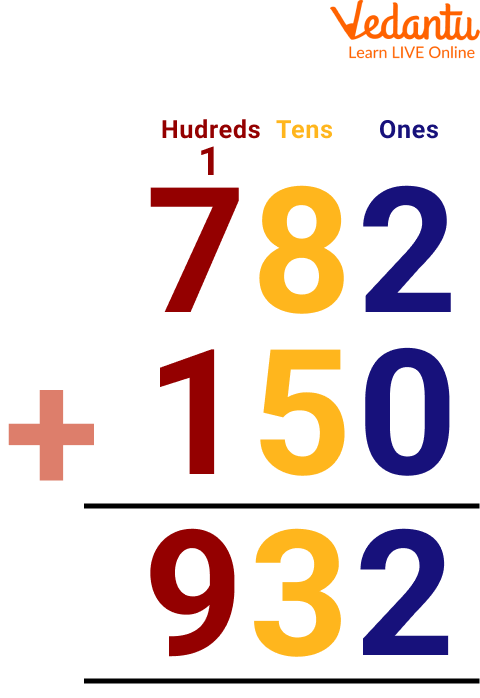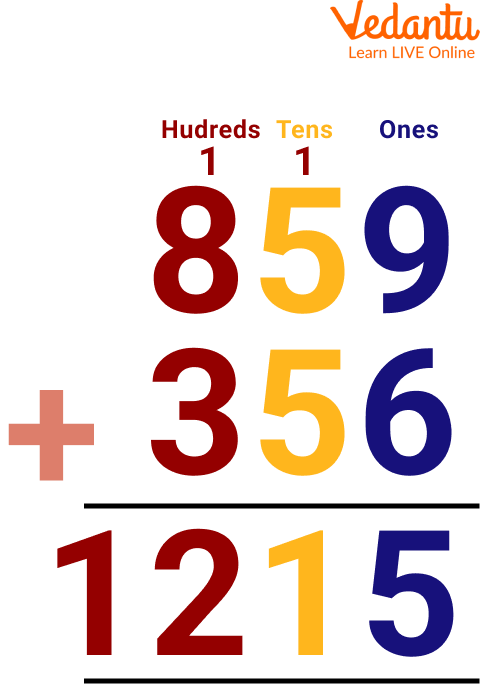Courses
Courses for Kids
Free study material
Free LIVE classes
More

# 3-Digit Addition Sums for Class 2## Introduction to 3-Digit Addition SumsWhen adding numbers, be sure that at least one addend or all of the addends contain three digits. By placing the three-digit numbers in columns according to their place values, we can add them. Then, after combining the numbers from the ones and tens columns, we go to the hundreds column. This process is termed as 3-digit addition.

Let us see the basic steps that are followed in 3-digit addition.

Step 1: Place the given numbers (addends) one below the other so that they fall correctly under the ones, tens, and hundreds columns.

Step 2: Start adding the numbers from right to left, beginning from the ones column, followed by the tens column and then the hundreds column.

Step 3: After all the columns are added, we get the numbers' sum.## Addition of 3-Digit Number with Carry

Regrouping is another name for carrying over. In addition, when the sum of the addends is greater than 9 in any of the columns, we regroup the numbers and carry over the extra digit to the preceding column. Let us understand this with the help of the following example, let us add 673 and 273.

### Let us understand 3-digit addition with regrouping using the following steps:

Step 1: Add the numbers in the ones column as a first step. 3 + 3 = 6. Then, in the ones column, enter 6.

Step 2: Add the numbers in the tens column in step two. 7 + 7 = 14. Place 1 in the hundreds column and 4 in the tens column. We took this step because adding 7 and 7 results in 14, but we are only allowed to write one digit beneath each column, so we cannot put 14 in the tens column. So, we add the number 14 together.

For this, we position the 4 in the matching column of tens, and the 1 on top of the column of hundreds (the preceding column). In other words, when we get two-digit integers as the total, we carry the tens digit to the next column to the left and place the ones digit in the corresponding column. This carry-over is included in the addition in the column where it is listed.

Step 3: Add the numbers in the hundreds column in step three. Thus, 6 + 2 + 1 (carryover) equals 9. The carry-over is added together with the other addends after being positioned in the hundreds column.

The result of adding 673 and 273 is 946.The sum of the numbers 673 and 273 is 946

## Add 2 and $\dfrac{2}{3}$

Below is the addition of 2 and $\dfrac{2}{3}$:

Given,

2 + $\dfrac{2}{3}$

$=\dfrac{2}{1}+\dfrac{2}{3}$

$=\dfrac{2 \times 3}{1 \times 3}+\dfrac{2}{3}$

Here we have equalized the denominator of both numbers.

$=\dfrac{6}{3}+\dfrac{2}{3}$

$=\dfrac{6+2}{3}$

$=\dfrac{8}{3}$

Thus the addition of 2 and $\dfrac{2}{3}$ gives the result of $\dfrac{8}{3}$.

## Solved Examples on Addition by Carry 3-Digit

Here are examples of addition with carry 3-digit, which are as follows:

Ans:Therefore, addition of 782 and 150 is 932.

Ans:Thus adding 859 to 356 gives 1215.

## Practice Questions

Here is given the addition of the 3-digit numbers worksheet:

Ans. 1197

Ans. 1011

Ans. 1269

Ans. 1197

Ans. 691

## Summary

3-digit addition is the addition of numbers here either one or all the addends are of 3 digits. We usually use the column addition method to add 3-digit numbers, where we place the given addends one below the other according to their place values and start adding them, beginning from the ones column, then moving on to the tens column and then the hundreds column. In this, we have also gone through the addition of a 3-Digit number with carry. In the end, we added some solved examples and practice problems to have better command over the topic.

Last updated date: 05th Jun 2023
Total views: 43.2k
Views today: 0.22k

## FAQs on 3-Digit Addition Sums for Class 2

1. Why is 3-digit addition important to learn?

3-digit addition is important to learn for kids because it assists them in learning the systematic way of adding the values. It also helps them master the concepts of place value. It will also help them in higher studies to calculate large figures.

2. When should a kid start learning addition?

The first math operations that children learn are addition and subtraction. However, it only occurs in stages. Students normally learn addition and subtraction in small steps between kindergarten and the fourth grade.

3. What is addition in class 3?

Combining things and counting them as one big group is done through addition. In math, addition is adding two or more integers together. Addends are the numbers added, while the total refers to the outcome of the operation.